AP State Syllabus AP Board 7th Class Maths Solutions Chapter 14 Understanding 3D and 2D Shapes InText Questions and Answers.

## AP State Syllabus 7th Class Maths Solutions 14th Lesson Understanding 3D and 2D Shapes InText QuestionsQuestion 1.
Estimate the number of cubes in the following arrangement. (Page No. 273)
Solution: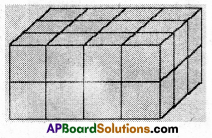4 × 3 × 2 = 24 No. of cubes in the above fig. = 24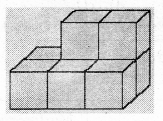3 × 2 + 2 = 6 + 2 = 8
No. of cubes in the above fig. = 8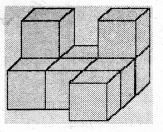3 + 2 + 2 + 1 + 1 = 9
No. of cubes in the above fig. = 9Question 2.
Two dice are placed side by side as shown. Can you say what the total would be on the faces opposite to them. (Page No. 274)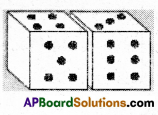i) 5 + 6 ii) 4 + 3
Solution:
i) Sum of the opposite faces = 2 + 1 = 3
ii) Sum of the opposite faces = 3 + 4 = 7

Question 3.
Three cubes each with 2 cm are placed side by side to form a cuboid. Try to make an oblique sketch and say what could be its length, breadth and height. (Page No. 274)
Solution: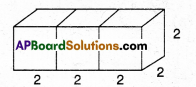Length =2 + 2 + 2 = 6cm
Height = 2 cmDo This

Question 1.
Make clay (or plasticine) models of the following solids and make vertical or horizontal cuts. Draw rough sketches of the cross-sections you obtain. Name them if possible. (Page No. 274)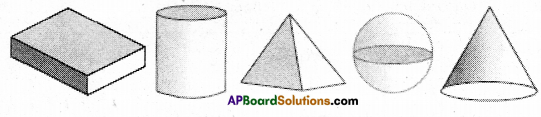Solution: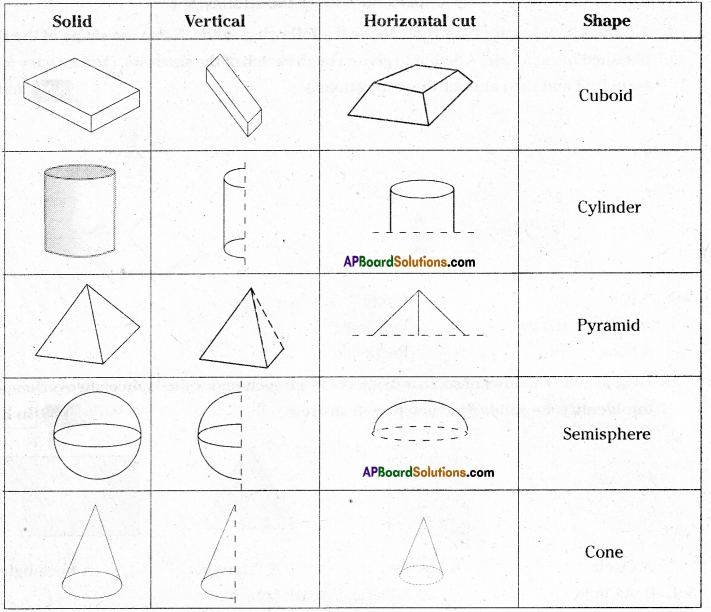Question 2.
What cross-section do you get when you give a i) vertical cut ii) horizontal cut to the following solids. (Page No. 275)
a) A brick
b) A round apple
c) A die
d) A circular pipe
e) An ice-cream cone
Solution:

 Solid Vertical cut Horizontal cut Brick Square/rectangle Rectangle A round apple Circle Circle Die Square Square Circular pipe Rectangle Circle ice-cream cone Triangle Circle